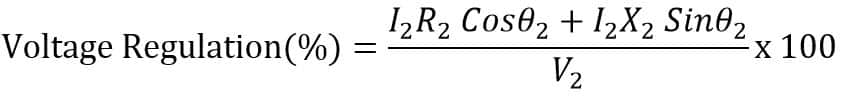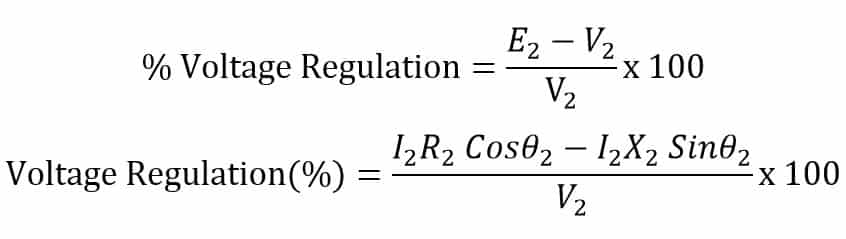# Voltage Regulation of Transformer-Definition,Formula

## What is Voltage Regulation of a Transformer?

Voltage regulation of transformer is defined as the percentage change in the transformer secondary voltage from no load to full load. In other words, the transformer voltage regulation describes the ability of the transformer to provide the constant voltage from no load to full load. The secondary voltage of the  transformer should not vary with load when the input supply is constant. The transformer can be treated as a voltage source and the variation in the secondary terminal voltage from no load to full load  depends on the voltage drop in the transformer winding.

When current flows through the transformer the voltage drop takes place due to reactance and resistance of the transformer.The variation in the secondary output voltage of the transformer  from its no load to full load  is called the voltage regulation of the transformer.

### Transformer Voltage Regulation Formula

The voltage regulation of the transformer shows how well a transformer maintains constant secondary output voltage from no load to full load condition when the primary voltage is constant.Where,
The secondary output voltage decrease as the current flowing through the transformer is increased.This happens  because of the voltage drop in the transformer winding. The secondary output voltage at full load is always less the the output voltage at no load.The lesser the voltage difference of  no load to full load condition, the better the regulation of the transformer.

#### Example of Voltage Regulation :

The transformer secondary output voltage is 220 volts at no load. The secondary voltage decrease from 220 to 210 volts when the transformer is loaded up to its full load current capacity.
The drop in the secondary output voltage from no load to full load = 10 VoltsIf the secondary voltage decrease from 220 to 215 volts when the transformer is loaded up to its full load current capacity. The regulation of the transformer in this case is 2.32 %. The % regulation of the transformer should be as minimum as possible to have almost constant secondary output voltage. Also the copper loss gets decreased when the regulation of the transformer is improved.

### What Factors affects the Transformer Voltage Regulation?

The following factors affects the voltage regulation of the transformer.

1. Resistance per phase – The resistance of the primary and secondary winding cause voltage drop. The more the resistance, the more the voltage drop. Transformer with higher primary and secondary resistance has poor voltage regulation.
2. Reactance per phase – The reactance of the primary and secondary winding cause voltage drop. The more the reactance, the more the voltage drop. Transformer with higher primary and secondary reactance has poor voltage regulation.
3. Leakage Flux – If leakage flux is more, the leakage reactance XL increases which increases Ia XL voltage drop. Hence regulation becomes poor.
4. Magnitude of secondary load current- If load current increases IaRa and IaXL voltage drop increases. Therefore terminals voltage drops which makes regulation poor.
5. Load Power factor : The load power factor also affects the transformer voltage regulation. The lagging power factor demands more current and it cause more voltage drop in transformer winding. As a result the voltage regulation of the transformer deteriorates. The leading power factor increase the transformer secondary voltage and thus the regulation of transformer improves.

The transformer have primary and the secondary winding. The winding has resistance and reactance. The primary resistance and reactance can be referred at the secondary side or vice versa. The  voltage regulation of the transformer depends on the reactance and the resistance of the transformer. The equivalent secondary circuit of the transformer is as given below.When the transformer is at no load, the secondary current I2 =0 and the transformer draws only no load current. The voltage drop I2Z2  across secondary impedance  takes place with an increase in the secondary current. The voltage drop in the secondary winding is maximum when transformer operates at its full kVA capacity delivering the rated secondary current to the load.
At no load the secondary terminal voltage = E2
At rated secondary current the voltage drop = I2Z2
At rated secondary current the terminal voltage = V2
According KCL,
E2   =  I2Z2 + V2
E2 – V2 =  I2Z2

The regulation of the transformer  depends on the power factor of the load. Now, we will discuss the regulation of the transformer at lagging, leading and unity power factor.

### Voltage Regulation of Transformer for Lagging Power Factor

Let the angle between the secondary  terminal voltage V2 and secondary current I2 is θ2. The phasor diagram of the no load voltage(E2 ) , full load voltage (V2) and current (I2)is as shown below.
From above diagram,
OC  = OA + AB + BC
In triangle ABE
AB = AE Cosθ2

In triangle DEF,

DC = DE Sinθ2

The angle between OC and OD is very small and OC is equal to OD.
OC = OD
OC = OA + AB + BC
E2  = V2  + AE Cosθ2 + DE Sinθ2
E2  = V2  + I2R2 Cosθ2 + I2X2 Sinθ2

E2  – V2  =  I2R2 Cosθ2 + I2X2 Sinθ2### Voltage Regulation of Transformer for Leading Power Factor

The phsor diagram of the transformer operating at leading power factor is as shown below.
From above diagram,
OC  = OA + AB – BC
In triangle ABE,
AB = AE Cosθ2
In triangle DEF,

BC = DE Sinθ2

The angle between OC and OD is very small and OC is equal to OD.

OC = OD
OC = OA + AB – BC

Here, OA = V2
E2  = V2  + AE Cosθ2 – DE Sinθ2
E2  = V2  + I2R2 Cosθ2 – I2X2 Sinθ2
E2  – V2  =  I2R2 Cosθ2 – I2X2 Sinθ2